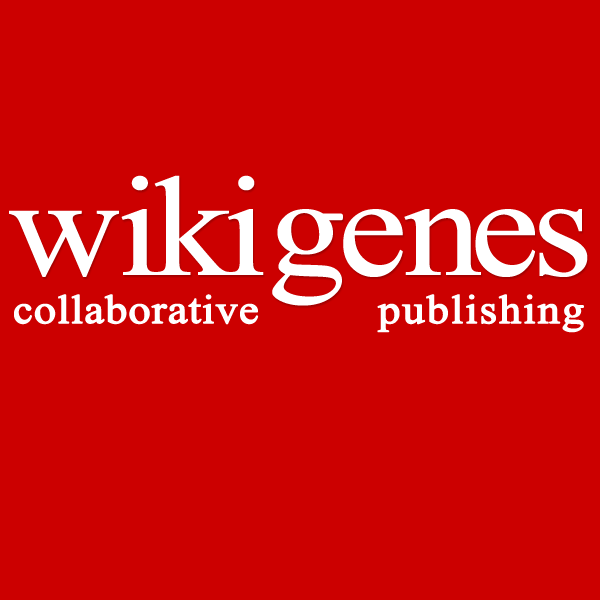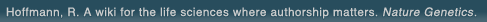# The world's first wiki where authorship really matters (Nature Genetics, 2008). Due credit and reputation for authors. Imagine a global collaborative knowledge base for original thoughts. Search thousands of articles and collaborate with scientists around the globe.

wikigene or wiki gene protein drug chemical gene disease author authorship tracking collaborative publishing evolutionary knowledge reputation system wiki2.0 global collaboration genes proteins drugs chemicals diseases compound
Hoffmann, R. A wiki for the life sciences where authorship matters. Nature Genetics (2008)# Cationic aluminum alkyl complexes incorporating aminotroponiminate ligands.

The synthesis, structures, and reactivity of cationic aluminum complexes containing the N,N'-diisopropylaminotroponiminate ligand ((i)Pr(2)-ATI(-)) are described. The reaction of ((i)Pr(2)-ATI)AlR(2) (1a-e,g,h; R = H (a), Me (b), Et (c), Pr (d), (i)Bu (e), Cy (g), CH(2)Ph (h)) with [Ph(3)C][B(C(6)F(5))(4)] yields ((i)()Pr(2)-ATI)AlR(+) species whose fate depends on the properties of the R ligand. 1a and 1b react with 0.5 equiv of [Ph(3)C][B(C(6)F(5))(4)] to produce dinuclear monocationic complexes [([(i)Pr(2)-ATI] AlR)(2)(mu-R)][(C(6)F(5))(4)] (2a,b). The cation of 2b contains two ((i)()Pr(2)-ATI)AlMe(+) units linked by an almost linear Al-Me-Al bridge; 2a is presumed to have an analogous structure. 2b does not react further with [Ph(3)C][B(C(6)F(5))(4)]. However, 1a reacts with 1 equiv of [Ph(3)C][B(C(6)F(5))(4)] to afford ((i Pr(2)-ATI)Al(C(6)F(5))(mu-H)(2)B(C(6)F(5))(2) (3) and other products, presumably via C(6)F(5)(-) transfer and ligand redistribution of a [((i)()Pr(2)-ATI)AlH][(C(6)F(5))(4)] intermediate. 1c-e react with 1 equiv of [Ph(3)C][B(C(6)F(5))(4)] to yield stable base-free [((i)Pr(2)-ATI)AlR][B(C(6)F(5))(4)] complexes (4c-e). 4c crystallizes from chlorobenzene as 4c(ClPh).0.5PhCl, which has been characterized by X-ray crystallography. In the solid state the PhCl ligand of 4c(ClPh) is coordinated by a dative PhCl-Al bond and an ATI/Ph pi-stacking interaction. 1g,h react with [Ph(3)C][B(C(6)F(5))(4)] to yield ((i)Pr(2)-ATI)Al(R)(C(6)F(5)) (5g,h) via C(6)F(5)(-) transfer of [((i)Pr(2)-ATI)AlR][(BC(6)F(5))(4)] intermediates. 1c,h react with B(C(6)F(5))(3) to yield ((i)Pr(2)-ATI)Al(R)(C(6)F(5)) (5c,h) via C(6)F(5)(-) transfer of [((i)Pr(2)-ATI)AlR][RB(C(6)F(5))(3)] intermediates. The reaction of 4c-e with MeCN or acetone yields [((i)Pr(2)-ATI)Al(R)(L)][B(C(6)F(5))(4)] adducts (L = MeCN (8c-e), acetone (9c-e)), which undergo associative intermolecular L exchange. 9c-e undergo slow beta-H transfer to afford the dinuclear dicationic alkoxide complex [(((i)Pr(2)-ATI)Al(mu-O(i)()Pr))(2)][B(C(6)F(5))(4)](2) (10) and the corresponding olefin. 4c-e catalyze the head-to-tail dimerization of tert-butyl acetylene by an insertion/sigma-bond metathesis mechanism involving [((i)Pr(2)-ATI)Al(C=C(t)Bu)][B(C(6)F(5))(4)] (13) and [((i)Pr(2)-ATI)Al(CH=C((t)()Bu)C=C(t)Bu)][B(C(6)F(5))(4)] (14) intermediates. 13 crystallizes as the dinuclear dicationic complex [([(i Pr(2)-ATI]Al(mu-C=C(t)Bu))(2)][B(C(6)F(5))(4)](2).5PhCl from chlorobenzene. 4e catalyzes the polymerization of propylene oxide and 2a catalyzes the polymerization of methyl methacrylate. 4c,e react with ethylene-d(4) by beta-H transfer to yield [((i)Pr(2)-ATI)AlCD(2)CD(2)H][B(C(6)F(5))(4)] initially. Polyethylene is also produced in these reactions by an unidentified active species.

## References

1. Cationic aluminum alkyl complexes incorporating aminotroponiminate ligands. Korolev, A.V., Ihara, E., Guzei, I.A., Young, V.G., Jordan, R.F. J. Am. Chem. Soc. (2001) [Pubmed]